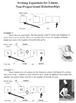# Reference - Integers, Expressions, Equations, InequalitiesSubject
Resource Type
Product Rating
4.0
5 Ratings
File Type

PDF (Acrobat) Document File

621 KB|9 pages
Share
Product Description
These reference pages cover integers, combining like terms, distribution, factoring, solving equations, and inequalities. I suggest letting students keep them on their desks while they work so you can simply refer them to the relevant section if they forget something rather than having to reteach it.

Negatives

1. This sheet breaks the rules for negative numbers down into three distinct sets: rewriting, adding and subtracting, and multiplying and dividing.
2. It shows how to use a number line or positive and negative circles to add or subtract.
3. It discusses the overall sign of a fraction based on the signs of the numerator and denominator.
4. As any middle school math teacher will know, students will often look at a problem like -99+23 and try to answer it by setting up a subtraction problem with 23 on the top and -99 on the bottom. This page also reminds students not to do that!

Finding the Distance between Two Points

1. Teaches students to use subtraction and absolute value when finding the distance between two points.
2. Explains that the order we subtract does not matter since we will be taking the absolute value anyway.
3. Reminds students that when they are subtracting a negative they should write both the minus sign and negative sign.

Combining Like Terms

1. Explains what like terms are, including terms with exponents and that combining them means to add or subtract.
2. Gives examples of like terms and unlike terms.
3. Explains that even if the variables in two terms are rearranged they could still be like terms because of the commutative property.
4. Reminds students that if they don't see a coefficient in front of a variable, it's 1.
5. Explains why unlike terms can't be combined.
6. Explains that more than two like terms may be combined.

Distribution and Factoring

1. Explains what distribution is and why it works using an area model.
2. Explains how to multiply the terms in one pair of parentheses with those of another.
3. Shows how to distribute within a larger expression.
4. Shows how to distribute just a plus or minus sign in front of the parentheses.
5. Discusses factoring as distribution in reverse.

Solving Equations

1. Reminds students that the goal of solving equations is to get the unknown quantity by itself.
2. Explains that inverse operations bring us back to the number we started with.
3. Explains that whatever is done to one side of an equation must also be done to the other side.
4. Shows students how to solve 10 very similar-looking (and easily confused) simple equations.

Solving Multi-Step Equations

1. While not an exhaustive account of how to solve equations, this page helps students plan their line of attack by thinking of "free" and "trapped" terms. Free terms may be removed immediately. Trapped terms, such as those inside parentheses or inside a fraction, must be freed before they can be removed.
2. Presents students with four different multi-step equations as well as their solutions.

Inequalities

1. Explains what inequality symbols are, referring to the "mouth" and "point" of the symbol as well as the line underneath.
2. Emphatically reminds students that inequality symbols are not arrows. This misunderstanding leads many students in the wrong direction when graphing inequalities.
3. Discusses reading inequalities from left to right or right to left.
4. Gives examples of solving inequalities and reminds students to flip the inequality symbol when multiplying or dividing by a negative number.
5. Explains how to graph inequalities.

Writing Equations for Proportional Relationships

1. Gives two examples of how a verbal description of a proportional situation may be modeled with an equation.

Writing Equations for Linear, Non-Proportional Relationships

1. Gives two examples of how a verbal description of a linear, non-proportional situation may be modeled with an equation.
Total Pages
9 pages